Deutsche VersionOhm's Law
Calculator and all Formulas

Resistance (ohms), current (amps), and voltage (volts)

 Ohm's Law is the linear proportionality between current and voltage that occurs for most conductors of electricity. A graph of voltage against current is a straight line. The gradient is the resistance.   Practitioners rarely speak of potential difference, when electrical voltage (drop) is meant. VIR

Electrical voltage = current times resistance "VIR"

 Input: current I      × amperes resistance R ohms Output: voltage V volts
 voltage V      ⁄ volts resistance R ohms current I amperes
 voltage V      ⁄ volts current I amperes resistance R ohms
= reset.

Formulas:          V = I × R          I = V / R          R = V / I

The mathematical formulas of Ohm's Law

Ohm's Law can be rewritten in three ways for calculating current, resistance, and voltage.
If a current I should flow through a resistor R, the voltage V can be calculated.
First Version of the (voltage) formula: V = I × R

If there is a voltage V across a resistor R, a current I flows through it. I can be calculated.
Second Version of the (current) formula: I = V / R

If a current I flows through a resistor, and there is a voltage V across the resistor. R can be calculated.
Third Version of the (resistance) formula: R = V / I

All of these variations of the so called "Ohm's Law" are mathematically equal to one another.

 Name Formula sign Unit Symbol voltage V or E volt V current I ampere (amp) A resistance R ohm Ω power P watt W

 What is the formula for electrical current? When the current is constant: I = Δ Q / Δ t I is the current in amps (A) Δ Q is the electric charge in coulombs (C), that flows at time duration of Δ t in seconds (s).   Voltage V = current I × resistance R   Power P = voltage V × current I

In electrical conductors, in which the current and voltage are proportional
to each other, ohm's law apply: V ~ I or VI = const.

Constantan wires or other metal wires held at a constant temperature meet well ohm's law.

"VI = R = const." ist not the law of ohm. It is the definition of the resistance.
Thereafter, in every point, even with a bent curve, the resistance value can be calculated.

For many electrical components such as diodes ohm's law does not apply.

"Ohm's Law" has not been invented by Mr. Ohm

 "U ⁄ I = R = const." is not the law of Ohm or Ohm's law. It is the definition of the resistance. Thereafter, in every point - even with a bent curve - the resistance value can be calculated. Ohm's law "postulates" following relationship: When a voltage is applied to an object, the electric current flowing through it changes the strength proportional to the voltage. In other words, the electrical resistance, defined as the quotient of voltage and currentis constant, and that is independent of voltage and current. The name of the law "honors" Georg Simon Ohm, who could prove this relationship for some simple electrical conductors as one of the first searchers. "Ohm's Law" has really not been invented by Ohm.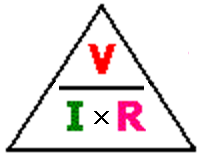Tip: Ohm's magic triangle The magic V I R  triangle can be used to calculate all formulations of ohm's law. Use a finger to hide the value to be calculated. The other two values then show how to do the calculation.
The symbol I or J = Latin: influare, international ampere, and R = resistance. V = voltage or
electric potential difference, also called voltage drop, or E = electromotive force (emf = voltage).

 Voltage drop calculations - DC / single phase calculation The voltage drop V in volts (V) is equal to the wire current I in amps (A) times twice the wire length L in feet (ft) times the wire resistance per 1000 feet R in ohms (Ω / kft) divided by 1000: Vdrop (V) = Iwire (A) × Rwire (Ω) = Iwire (A) × (2 × L (ft) × Rwire (Ω / kft) / 1000 (ft / kft))   The voltage drop V in volts (V) is equal to the wire current I in amps (A) times twice the wire length L in meters (m) times the wire resistance per 1000 meters R in ohms (Ω / km) divided by 1000: Vdrop (V) = Iwire (A) × Rwire (Ω) = Iwire (A) × (2 × L (m) × Rwire (Ω / km) / 1000 (m / km))

If the unit of power P = I × V and of voltage V = I · R is needed,
look for"The Big Power Formulas":
Calculations: power (watt), voltage, current, resistance

Some persons think that Georg Simon Ohm calculated the "specific resistance".
Therefore they think that only the following can be the true ohm's law.

 Quantity of resistanceR = resistance Ω ρ = specific resistance Ω×m l = double length of the cable m A = cross section mm2

Electrical conductivity (conductance) σ (sigma) = 1/ρ
Specific electrical resistance (resistivity) ρ (rho) = 1/σ

 Electrical  conductor Electrical conductivity  Electrical conductance Electrical resistivity  Specific resistance silver σ = 62 S·m/mm² ρ = 0.0161 Ohm∙mm²/m copper σ = 58 S·m/mm² ρ = 0.0172 Ohm∙mm²/m gold σ = 41 S·m/mm² ρ = 0.0244 Ohm∙mm²/m aluminium σ = 36 S·m/mm² ρ = 0.0277 Ohm∙mm²/m constantan σ = 2.0 S·m/mm² ρ = 0.5000 Ohm∙mm²/m

Difference between electrical resistivity and electrical conductivity

 The conductance in siemens is the reciprocal of the resistance in ohms.

 Simply enter the value to the left or the right side. The calculator works in both directions of the ↔ sign.
 Electrical conductivity σ    S · m / mm² ↔ Specific elec. resistance ρ    Ohm ∙ mm² / m σ = 1 / ρ ρ = 1 / σ siemens S = 1/Ω or ohm Ω = 1/S
 The value of the electrical conductivity (conductance) and the specific electrical resistance (resistivity) is a temperature dependent material constant. Mostly it is given at 20 or 25°C. Resistance R = ρ × (l / A) or R = l / (σ × A)

 For all conductors the specific resistivity changes with the temperature. In a limited temperature range it is approximately linear: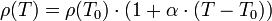where α is the temperature coefficient, T is the temperature and T0 is any temperature, such as T0 = 293.15 K = 20°C at which the electrical resistivity ρ (T0) is known.

Cross-sectional area - cross section - slice plane

Now there is the question:
How can we calculate the cross sectional area (slice plane) A
from the wire diameter d and vice versa?

Calculation of the cross section A (slice plane) from diameter d: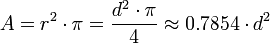r = radius of the wire
d = diameter of the wire

Calculation diameter d from cross section A (slice plane):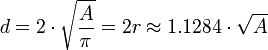Cross section A of the wire in mm2 inserted in this formula gives the diameter d in mm.

Calculation − Round cables and wires:
• Diameter to cross section and vice versa •

Electric voltage V = I × R      (Ohm's law VIR)
Electrical voltage = amperage × resistance (Ohm's law)
Please enter two values, the third value will be calculated.

 Electric voltage V volts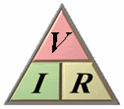Amperage I amps Resistance R ohms V = I × R              I = V / R              R = V / I
Electric power P = I × V      (Power law PIV)
Electric power = amperage × voltage (Watt's Law)
Please enter two values, the third value will be calculated.

 Electric Power P watts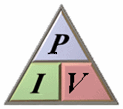Amperage I amps Voltage V volts P = I × V              I = P / V              V = P / I
 Ohm's law. V = I × R, where V is the potential across a circuit element, I is the current through it, and R is its resistance. This is not a generally applicable definition of resistance. It is only applicable to ohmic resistors, those whose resistance R is constant over the range of interest and V obeys a strictly linear relation to I. Materials are said to be ohmic when V depends linearly on R. Metals are ohmic so long as one holds their temperature constant. But changing the temperature of a metal changes R slightly. When the current changes rapidly, as when turning on a light, or when using AC sources, slightly non-linear and non-ohmic behavior can be observed. For non-ohmic resistors, R is current-dependent and the definition R = dV/dI is far more useful. This is sometimes called the dynamic resistance. Solid state devices such as thermistors are non-ohmic and non-linear. A thermistor's resistance decreases as it warms up, so its dynamic resistance is negative. Tunnel diodes and some electrochemical processes have a complicated I to V curve with a negative resistance region of operation. The dependence of resistance on current is partly due to the change in the device's temperature with increasing current, but other subtle processes also contribute to change in resistance in solid state devices.

 How electricity works.   Ohm's Law clearly explained.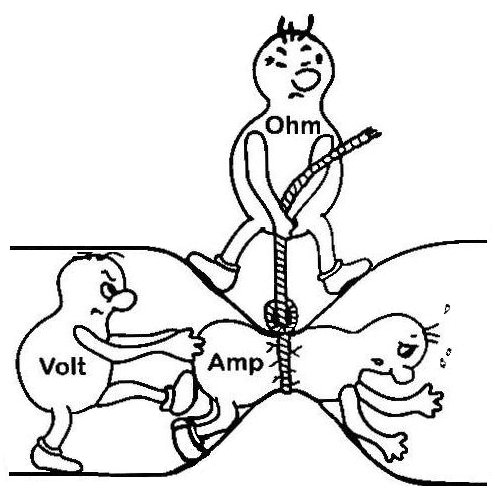[top of page]